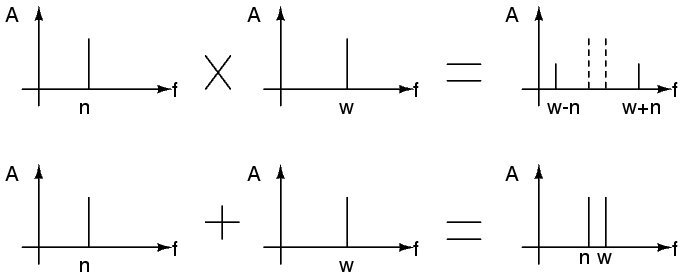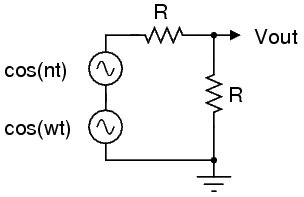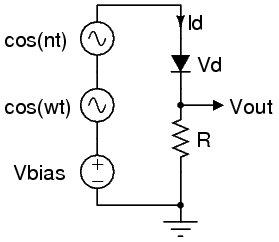2007-12-31

# Non-Linearity, Harmonic Generation and Frequency Mixing

This article is inspired by SolderSmoke 72, where Bill states that he understands the multiplication identity maths, but not the actual physical mechanism of frequency mixing through non-linear circuit elements. I decided to try to answer that question, to myself mainly, to deepen my own understanding. Of course, it is also an excuse to use the new MathML support!

As Bill said, frequency mixing results from multiplication of two sinusoids, the familiar trig identity that most of us were forced to memorise at school shows this:

`cos(omega t)*cos(nu t) = (cos((omega-nu)t) + cos((omega + nu)t))/2`

A graphical example might be:

times gives agraph width=200; height=100; axes(); plot(cos(4x)); text((3,-2), "`cos(4x)`"); endgraph agraph width=200; height=100; axes(); plot(cos(5x)); text((3,-2), "`cos(5x)`");endgraph agraph width=200; height=100; axes(); plot(cos(4x)*cos(5x)); text((3,-2), "`cos(4x).cos(5x)`"); endgraph agraph width=200; height=100; axes(); plot(cos(x)/2); text((3,-2), "`cos(x)/2`");endgraph agraph width=200; height=100; axes(); plot(cos(9x)/2); text((3,-2), "`cos(9x)/2`");endgraph

An aside to electronics for a moment: This is what we might see at the output of a perfect four-quadrant multiplier using a CRO. This might be confusing to some, compared to say the output of a DSB modulator driven with an pure AF tone (which looks like what we see below). However remember than the DSB signal is actually the superposition of two RF tones, the sum and difference frequency, with the AF and carrier suppressed. So quite rightly it looks like the beating below because it actually is the sum of two RF tones. For an SSB signal you'd see just the one constant RF tone as one product is suppressed/filtered. For a two-tone test you'd see the beating again, and a more complex 4-tone superposition for a two-tone DSB signal.

Simple addition (superposition) on the other hand, produces the characteristic "beating" pattern, but this is not frequency mixing. The components are simply superimposed. For perfectly linear systems no mixing products are produced at all, despite the appearance of the addition identity and what we might see on the CRO:

`cos(omega t) + cos(nu t) = 2 cos(((omega + nu)/2)t) cos(((omega - nu)/2)t)`

A graphical example:

plus gives agraph width=200; height=100; axes(); plot(cos(4x)); text((3,-2), "`cos(4x)`"); endgraph agraph width=200; height=100; axes(); plot(cos(5x)); text((3,-2), "`cos(5x)`"); endgraph agraph width=200; height=100; axes(); plot(cos(4x)+cos(5x)); text((3,-2), "`cos(4x)+cos(5x)`"); endgraph agraph width=200; height=100; axes(); plot(cos((9/2)x)); text((3,-2), "`cos((9/2)x)`"); endgraph agraph width=200; height=100; axes(); plot(cos((1/2)x)); text((3,-2), "`cos((1/2)x)`"); endgraph

The envelope of the `(omega - nu)/2` component is clearly visible, but a filter can't extract energy at `(omega - nu)/2` because there is none there, neither is there energy at `(omega + nu)/2`. Filters at `omega` or `nu` would however recover the individual components. This is obvious if we use our first multiplicative identity upon each apparent "component", essentially reversing the process and giving us the separate `omega` and `nu` tones back again. You might like to think of the sum of two tones as that which would result from the multiplication of tones of `sqrt(2)` amplitude at their average and half their difference. This is somewhat intuitive if you think of it as the result of DSB modulating `sqrt(2)cos(((omega + nu)/2)t)` with `sqrt(2)cos(((omega - nu)/2)t)` which is `cos(omega t) + cos(nu t)`.

## In the Frequency Domain

I personally find it somewhat confusing to visualise this in the time domain, so here is a frequency domain picture of what is going on:## Multiplication from Non-Linearity

Lets first consider a linear circuit; two resistors `R` fed from two AC voltage sources operating at `omega` and `nu` radians per second, delivering 1 volt pk-pk each.The transfer function is perfectly linear, the output voltage is one half the input voltage, and the input voltage is the simple sum of the instantaneous voltages of each AC source.

`V_(out) = 1/2 (cos(omega t) + cos(nu t))`

From our identity investigation above, this contains only `omega` and `nu` components in the frequency domain. No mixing products are produced.

Now lets replace the series resistor with a diode.The diode is the classic non-linear component, its dynamic properties described by the Shockley diode equation:

`I_d = I_s(e^(V_d/(nV_T)) - 1)`

Unfortunately this is absolutely horrible to solve analytically, and I won't attempt to do so here. Read the wikipedia Diode Modelling article for a starting point on the gory details. Instead, I'll just assume the diode gives as an exponentially changing "resistance" with the applied voltage. This is sufficient for a hand-waving argument to show how multiplication occurs.Consider `cos(omega t) + cos(nu t)` being exponentiated by the diode `V_d`/`I_d` characteristic. Assume `nu` is much smaller than `omega`, such that we can consider `cos(nu t)` to be almost DC during cycles of `cos(omega t)`. We'll also assume there is a DC bias that prevents the diode rectifying the signals and they are of sufficiently small amplitude to be operating near the "knee" of the diode curve - this simplifies the argument and helps the visualisation, but isn't strictly required. The voltage seen across the resistor varies something like `e^(A cos(omega t) + k)`. Where `k` is current value of `cos(nu t)` and `A` some small amplitude constant. You can visualise this as `cos(nu t)` slowly sliding the `cos(omega t)` up and down the diode `V_d`/`I_d` curve. Towards the bottom `I_d` varies quite slowly with `V_d`, so `V_(out)` is some small almost constant factor of (a distorted) `cos(omega t)`, further up this "constant" increases as the slope of the `V_d`/`I_d` curve increases and so the amplitude of the passed `cos(omega t)` increases. You can think of it as `cos(nu t)` modulating the diode's dynamic resistance and hence multiplying its instantaneous value with `cos(omega t)`.

Actually both voltages affect each other, and the products produced also mix. This is intermodulation distortion.

All this non-linearity also causes harmonic distortion of singly applied signals, distorting their waveshape and pushing some of their energy into harmonics. In the simple diode example all kinds of junk is produced, with them all intermodulating each other right down into the noise floor.

## More Rigor

I am always troubled by hand-waving arguments, even if they provide some intuitive understanding. Quite frequently such "lies to children" are just that, complete falsehoods of dubious pedagogical merit. Here is a more mathematically sound argument...

Consider the Taylor expansion of `e^x`:

`e^x = sum_(n=0)^oo(x^n/(n!))`

It is therefore easy to show that `e^x - 1` is well approximated by `x + x^2/2` for small `x`. Remembering for the diode equation our `x` here will contain `V_d` and that `(a + b)^2 = a^2 + 2ab + b^2` it is easy to see that there will be an `a*b` term in the transfer function somewhere. When `a` and `b` are our sinusoids `x = a+b` and we have our multiplying action!

This is the very heart of intermodulation through a diode.

The other higher-order terms are less important because their denominator grows as `n!` and will soon be into the noise floor, but it is helpful to remember they exist. In RF systems the 3rd-order products are of some concern, because they are quite close to the signals of interest. 3rd-order intercept is hence a figure of merit for high-performance mixers.

This same expansion explains harmonic distortion if you consider that `cos^2(omega t) = (1 + cos(2 omega t))/2` and that `cos^n(omega t)` has a `cos(n omega t)` term in general.

It is possible to show that all non-linear systems will produce harmonics and intermodulation products. The phase and amplitude of the products themselves are a function of the particular system, only in perfectly linear systems do the higher-order terms vanish.

## Multiplication by Switching

Switching mixers can be thought of as multiplying the signal by `+k` or `-k` on each excursion of the local oscillator waveform. Selectively inverting the signal at the LO rate. Basically the LO is a square wave and its harmonic comb is convolved with the input signal, resulting in a heap of mixing products around each harmonic as well as the fundamental. Filtering is usually used to pick the IF desired, but this also gives an idea how harmonic and sub-harmonic mixing works.

Symmetric `+-k` multiplication is not strictly required, even just chopping the signal on and off at the LO rate is sufficient to achieve frequency mixing (but suffers from other problems due to lack of balance). This can be visualised as sampling the signal at the LO rate, where the IF is an alias. If you remove the `V_(bias)` from our diode example above you have an unbalanced product detector which functions essentially by switching (the alias being at baseband). In practice it would need to be driven fairly hard by the LO and would tend to suffer from AM break-through, however such circuits are used after IF filtering in many receivers as the signals presented to it are well controlled.

Although not normally described as mixing, the rectification often used to demodulate full-carrier AM is also an example of mixing by switching. The carrier toggles the rectified path on and off at the carrier rate, mixing itself with the sidebands also present and generating the baseband image (basically folding both sidebands over each other).

True four-quadrant multipliers are ideal mixers and the LO signal remains as pure as it was injected. "Gilbert Cell" mixers approach this, and also suppress the injected LO and RF signals because of their symmetry. Leaving mostly the IF (and the image) at the output, simplifying the filtering of the IF.

## Parametric Mixing

We've only discussed time-varying resistance causing intermodulation here, but it is possible to vary reactance as well (and impedance in general). Parametric mixers use their pump waveform (LO) to alter the reactance of tuned circuits at the LO rate. One or more tuned circuits may be used, depending on the desired result.

In a parametric mixer one tuned circuit is set up to resonate at the desired IF, and other at the signal frequency, they are coupled through a varactor which is then pumped at the sum or difference, converting energy at the signal rate into that of the IF rate. The actual mechanism can be considered energy shunting between the coupled resonators as their LCR properties are varied, but the mathematics is pretty ugly. Double-sideband parametric mixers have three resonators, allowing extraction of both the sum and difference IFs. The IF resonators are generally called the idler, which I assume is a term from their mechanical origin?

It is possible to use a parametric mixer as an amplifier. Parametric mixers have gain as the pump energy is converted into the signal and IF frequencies. Hence a small signal in the signal resonator experiences amplification and can be extracted there if so desired (directional couplers/circulators are useful to isolate the input and output). The gain of the amplifier is controlled by varying the amplitude of the pump. Pump too hard and you simply have a parametric oscillator at the IF and signal frequencies.

Degenerate parametric amplifiers have only a single resonator (i.e. their IF equals the signal frequency) and are pumped at exactly twice their resonance frequency. This makes them quite phase-sensitive as the pumping must be coherent. The pump frequency for any parametric device must be at least twice the signal frequency.

Parametric mixing and amplification is typically used at very high frequencies, usually microwaves up to light. It is an extremely common practice in optics and low noise microwave converters. However, it can in principle be used at any frequency, and even in non-electronic systems, such as mechanics. The playground swing is a familiar example to all of us, changing your centre of mass dynamically alters the inertial terms of the system and pumps the oscillation (degenerate parametric amplification).

1 comment.

## Attachments

title type size
Linear System Diagram Source application/postscript 9.722 kbytes
Non-Linear System Diagram Source application/postscript 10.569 kbytes
"Non-Linear Multiplication" Diagram Source application/postscript 9.788 kbytes
The Frequency Domain Picture Source application/postscript 10.181 kbytes